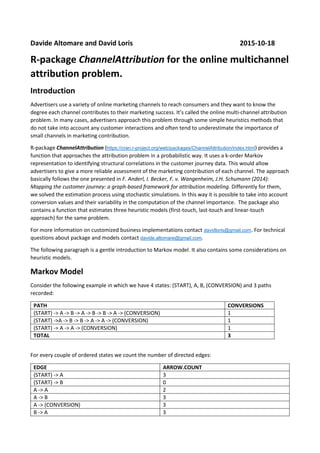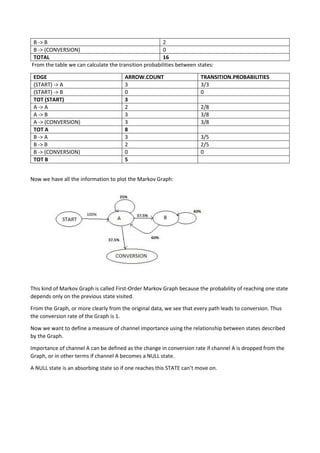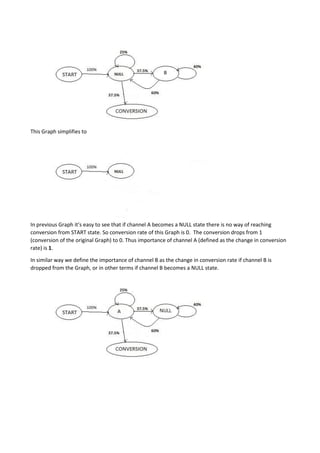Successfully reported this slideshow.

# Markov model for the online multichannel attribution problem

48

Share×
1 of 7
1 of 7

# Markov model for the online multichannel attribution problem

48

Share

## More Related Content

### Related Books

Free with a 14 day trial from Scribd

See all

### Related Audiobooks

Free with a 14 day trial from Scribd

See all

### Markov model for the online multichannel attribution problem

1. 1. Davide Altomare, David Loris 2016-12-07 R-package ChannelAttribution for the online multichannel attribution problem. Introduction Advertisers use a variety of online marketing channels to reach consumers and they want to know the degree each channel contributes to their marketing success. It’s called the online multi-channel attribution problem. In many cases, advertisers approach this problem through some simple heuristics methods that do not take into account any customer interactions and often tend to underestimate the importance of small channels in marketing contribution. R-package ChannelAttribution (https://cran.r-project.org/web/packages/ChannelAttribution/index.html) provides a function that approaches the attribution problem in a probabilistic way. It uses a k-order Markov representation to identifying structural correlations in the customer journey data. This would allow advertisers to give a more reliable assessment of the marketing contribution of each channel. The approach basically follows the one presented in F. Anderl, I. Becker, F. v. Wangenheim, J.H. Schumann (2014): Mapping the customer journey: a graph-based framework for attribution modeling. Differently for them, we solved the estimation process using stochastic simulations. In this way it is possible to take into account conversion values and their variability in the computation of the channel importance. The package also contains a function that estimates three heuristic models (first-touch, last-touch and linear-touch approach) for the same problem. The following paragraph is a gentle introduction to Markov model. It also contains some considerations on heuristic models. Markov Model Consider the following example in which we have 4 states: (START), A, B, (CONVERSION) and 3 paths recorded: PATH CONVERSIONS (START) -> A -> B -> A -> B -> B -> A -> (CONVERSION) 1 (START) ->A -> B -> B -> A -> A -> (CONVERSION) 1 (START) -> A -> A -> (CONVERSION) 1 TOTAL 3 For every couple of ordered states we count the number of directed edges: EDGE ARROW.COUNT (START) -> A 3 (START) -> B 0 A -> A 2 A -> B 3 A -> (CONVERSION) 3 B -> A 3 B -> B 2
2. 2. B -> (CONVERSION) 0 TOTAL 16 From the table we can calculate the transition probabilities between states: EDGE ARROW.COUNT TRANSITION.PROBABILITIES (START) -> A 3 3/3 (START) -> B 0 0 TOT (START) 3 A -> A 2 2/8 A -> B 3 3/8 A -> (CONVERSION) 3 3/8 TOT A 8 B -> A 3 3/5 B -> B 2 2/5 B -> (CONVERSION) 0 0 TOT B 5 Now we have all the information to plot the Markov Graph: This kind of Markov Graph is called First-Order Markov Graph because the probability of reaching one state depends only on the previous state visited. From the Graph, or more clearly from the original data, we see that every path leads to conversion. Thus the conversion rate of the Graph is 1. Now we want to define a measure of channel importance using the relationship between states described by the Graph. Importance of channel A can be defined as the change in conversion rate if channel A is dropped from the Graph, or in other terms if channel A becomes a NULL state. A NULL state is an absorbing state so if one reaches this STATE can’t move on.
3. 3. This Graph simplifies to In previous Graph it’s easy to see that if channel A becomes a NULL state there is no way of reaching conversion from START state. So conversion rate of this Graph is 0. The conversion drops from 1 (conversion of the original Graph) to 0. Thus importance of channel A (defined as the change in conversion rate) is 1. In similar way we define the importance of channel B as the change in conversion rate if channel B is dropped from the Graph, or in other terms if channel B becomes a NULL state.
4. 4. This Graph simplifies to: In previous Graph we see the probability of reaching conversion from START state is 0.5. Conversion drops from 1 (conversion rate of the original Graph) to 0.5. Thus the importance of channel B (defined as the change in conversion rate) is 0.5. Once we have the importance weights of every channel we can do a weighted imputation of total conversions (3 in this case) between channels. CHANNEL CONVERSIONS A 2 [ =3 x 1/(1+0.5) ] B 1 [ = 3 x 0.5/(1+0.5) ] TOTAL 3 Now let’s go back to the original data: PATH CONVERSIONS (START) -> A -> B -> A -> B -> B -> A -> (CONVERSION) 1 (START) ->A -> B -> B -> A -> A -> (CONVERSION) 1 (START) -> A -> A -> (CONVERSION) 1 TOTAL 3 We see that if we use a first-touch or last-touch approach, all the conversions are assigned to channel A, despite the important work of channel B in “conversion game” is clear from the data. The channel attribution problem can be viewed as a football match, to better understand how different approaches work. So channels can be viewed as players, paths are game actions and conversions are goals. Markov Model analyses relationships between game actions to understand the role of the player in scoring. While heuristic approach analyzes one action (path) at the time. So last-touch approach rewards only players who scored, while first-touch approach rewards only players who started the action. Linear approach rewards with the same credit to every player who took part the action, while time-decay approaches gives subjective weights to every player who took part the action. As we have seen Markov Model require as inputs paths and total conversions and does not require subjective assumptions, differently from heuristic approaches.
5. 5. Package ChannelAttribution In the following example we will show how ChannelAttribution can be used for the multichannel attribution problem. Load libraries and data: library(ChannelAttribution) library(reshape2) library(ggplot2) data(PathData) Estimate heuristic models: H=heuristic_models(Data,'path','total_conversions',var_value='total_conversion_value') Estimate Markov model: M=markov_model(Data, 'path', 'total_conversions', var_value='total_conversion_value') Plot total conversions: R=merge(H,M,by='channel_name') R1=R[,(colnames(R)%in%c("channel_name","first_touch_conversions","last_touch_conversions","linear _touch_conversions","total_conversion"))] colnames(R1)=c('channel_name','first_touch','last_touch','linear_touch','markov_model') R1=melt(R1,id="channel_name") ggplot(R1, aes(channel_name, value, fill = variable)) + geom_bar(stat="identity", position = "dodge") + ggtitle("TOTAL CONVERSIONS")+ theme(axis.title.x = element_text(vjust=-2))+ theme(axis.title.y = element_text(vjust=+2))+ theme(title = element_text(vjust=2))+ theme(text = element_text(size=16)) + theme(plot.title=element_text(size=20)) + ylab("")
6. 6. Plot total value: R2=R[,(colnames(R)%in%c("channel_name","first_touch_value","last_touch_value","linear_touch_value ","total_conversion_value"))] colnames(R2)=c('channel_name','first_touch','last_touch','linear_touch','markov_model') R2=melt(R2,id="channel_name") ggplot(R2, aes(channel_name, value, fill = variable)) + geom_bar(stat="identity", position = "dodge") + ggtitle("TOTAL VALUE")+ theme(axis.title.x = element_text(vjust=-2))+ theme(axis.title.y = element_text(vjust=+2))+ theme(title = element_text(vjust=2))+ theme(text = element_text(size=16)) + theme(plot.title=element_text(size=20)) + ylab("") ChannelAttribution Tool ChannelAttribution can be used through a user-friendly graphical interface without interfacing with R-code. Channel Attribution Tool can be found at https://adavide1982.shinyapps.io/ChannelAttribution. ChannelAttribution Tool can be also used locally through package ChannelAttributionApp. library(ChannelAttributionApp) CAapp()